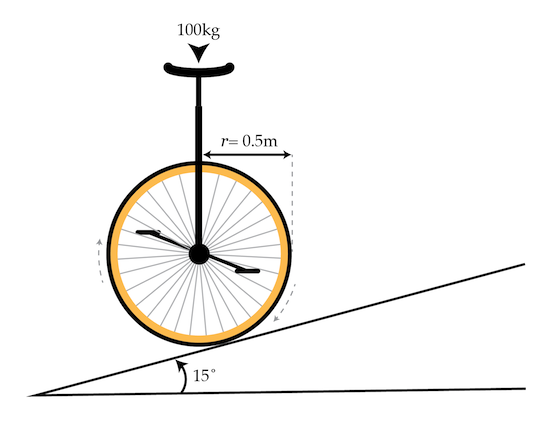# Better not stop pedaling

Between doing physics problems on Brilliant, some people like to unicycle. A unicyclist is cycling up a hill angled $15^\circ$ with respect to the horizontal. The center of mass of the cyclist is directly over the axle of the wheel and the cyclist/unicycle system have a combined mass of $\SI{100}{\kilo\gram}.$ The radius of the wheel is $\SI{0.5}{\meter}$ and the coefficient of static friction between the wheel and the asphalt is $1.$

What is the magnitude of the torque (in $\si{\newton\meter}$) that the cyclist needs to exert on the pedals in order to cycle up the hill at a constant speed?Details and assumptions

• The unicycle does not slip against the hill.
• You may take the acceleration of gravity to be $-\SI[per-mode=symbol]{9.8}{\meter\per\second\squared}.$
• You may neglect air resistance.
×

Problem Loading...

Note Loading...

Set Loading...